# Preparation of Sodium Hydroxide Titrant: Prepare 500 mL of a 0.1 M sodium hydroxide solution by...

Preparation of Sodium Hydroxide Titrant: Prepare 500 mL of a 0.1 M sodium hydroxide solution by dilution of a 1 M sodium hydroxide stock reagent or from solid NaOH. This dilution need only be performed to 5% accuracy, that is, using a graduated cylinder and an Erlenmeyer flask. Store the diluted solution in a polyethylene bottle. Standardize the diluted solution against dry, solid KHP (potassium hydrogen phthalate) using phenolphthalein as an indicator and label the bottle with the concentration of sodium hydroxide together with the accuracy (as represented by the 95% confidence limit).

Please explain the purpose of standardization. After standardization, a titration was performed with the above titrant and the analyte was KHP with unknown concentration. Please explain why it was important to standardize before performing the next titration.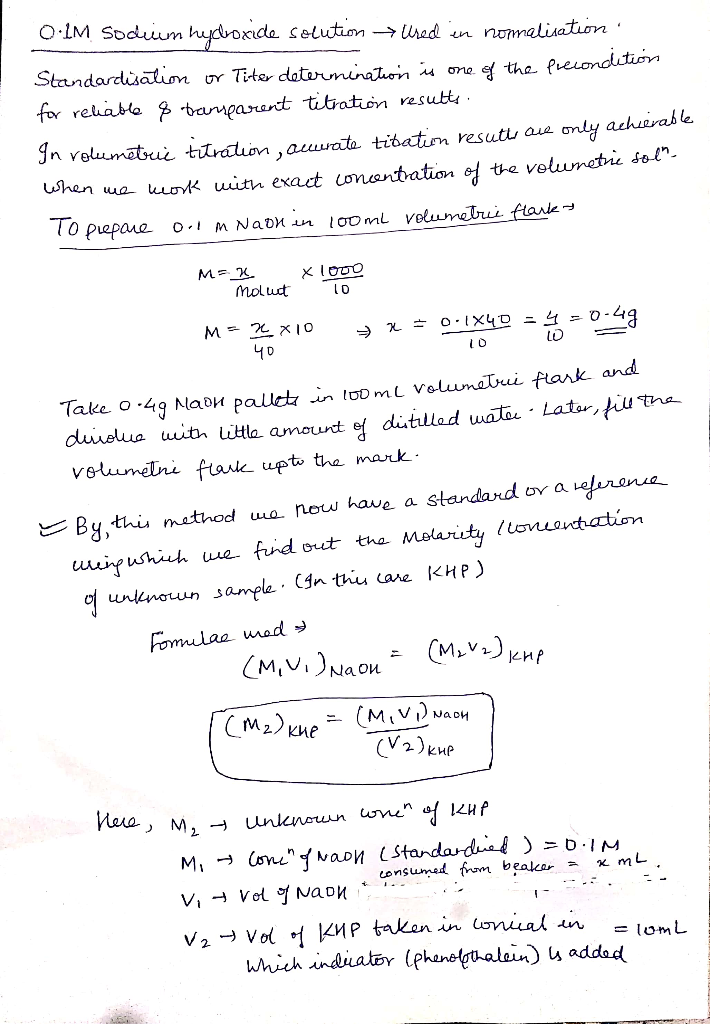#### Earn Coin

Coins can be redeemed for fabulous gifts.

Similar Homework Help Questions
• ### "Prepare 500 mL of a 0.1 M sodium hydroxide solution by dilution of a 1 M...

"Prepare 500 mL of a 0.1 M sodium hydroxide solution by dilution of a 1 M sodium hydroxide stock reagent or from solid NaOH. This dilution need only be performed to 5% accuracy, that is, using a graduated cylinder and an Erlenmeyer flask." 1. Explain why it is not necessary and even not desirable to perform the preparation of 1 M sodium hydroxide operation with higher accuracy. "Add the analyte to the beaker and fill with distilled deionized water to...

• ### KHP, potassium hydrogen phthalate (KHCHO is often used to standardie basic solution used in titration. If...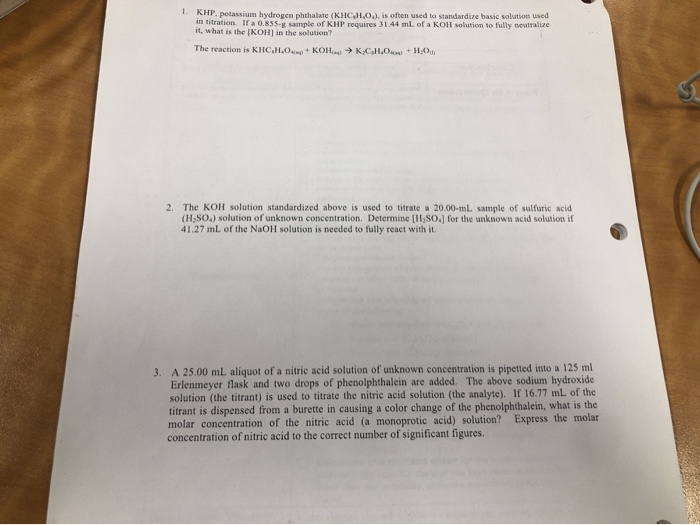KHP, potassium hydrogen phthalate (KHCHO is often used to standardie basic solution used in titration. If a 0.855. sample or KHP requires 31.44 ml of a KOR solution to fully realize it, what is the (KOH) in the solution? The reaction is KHC.H.O. KOHK C HO H O . 2. The KOH solution standardized above is used to titrate a 20.00-ml sample of sulfuric acid (H,SO.) solution of unknown concentration. Determine (H.SO.) for the unknown acid solution if 41.27 mL...

• ### 1. A solution of sodium hydroxide (NaOH) was standardized against potassium hydrogen phthalate (KHP). A known mass...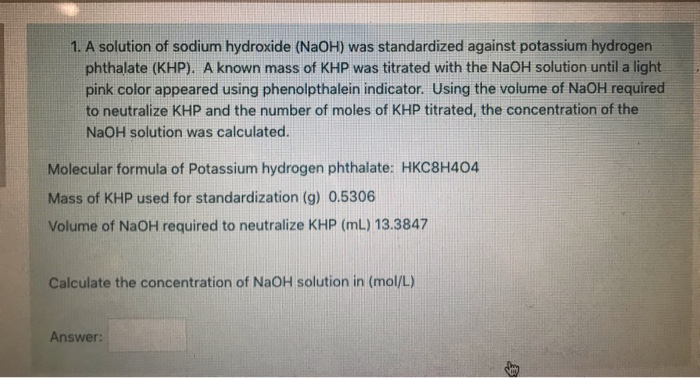1. A solution of sodium hydroxide (NaOH) was standardized against potassium hydrogen phthalate (KHP). A known mass of KHP was titrated with the NaOH solution until a light pink color appeared using phenolpthalein indicator. Using the volume of NaOH required to neutralize KHP and the number of moles of KHP titrated, the concentration of the NaOH solution was calculated. Molecular formula of Potassium hydrogen phthalate: HKC8H404 Mass of KHP used for standardization (g) 0.5306 Volume of NaOH required to neutralize...

• ### 1. A student is asked to standardize a solution of sodium hydroxide. He weighs out 0.960...

1. A student is asked to standardize a solution of sodium hydroxide. He weighs out 0.960 g potassium hydrogen phthalate (KHC8H4O4, treat this as a monoprotic acid). It requires 18.1 mL of sodium hydroxide to reach the endpoint. A. What is the molarity of the sodium hydroxide solution? ___M This sodium hydroxide solution is then used to titrate an unknown solution of hydroiodic acid. B. If 12.8 mL of the sodium hydroxide solution is required to neutralize 27.8 mL of...

• ### a Saturated solution of magnesium hydroxide is prepared and the excess solid magnesium hydroxide is allowed to settle....

a Saturated solution of magnesium hydroxide is prepared and the excess solid magnesium hydroxide is allowed to settle. A 25ml aliquot of the saturated solution is withdrawn and transferred to an Erlenmeyer flask, and two drops of methyl orange indicator are added. A 0.00053 M HCl solution(titrant) is dispensed from a buret into the solution (analyte). The solution turns from yellow to a very faint red-orange after the addition of 13.2ml a. How many moles of hydroxide ion are neutralized...

• ### What is the concentration of a sodium hydroxide solution if 0.2033 g of potassium hydrogen phthalate...

What is the concentration of a sodium hydroxide solution if 0.2033 g of potassium hydrogen phthalate (KHP- MW 204.22 g/mol) requires 10.40 mL of NaOH solution to reach the phenolphthalein end point? Answer with three decimal places.

• ### 1. A solution of sodium hydroxide (NaOH) was standardized against potassium hydrogen phthalate (KHP). A known...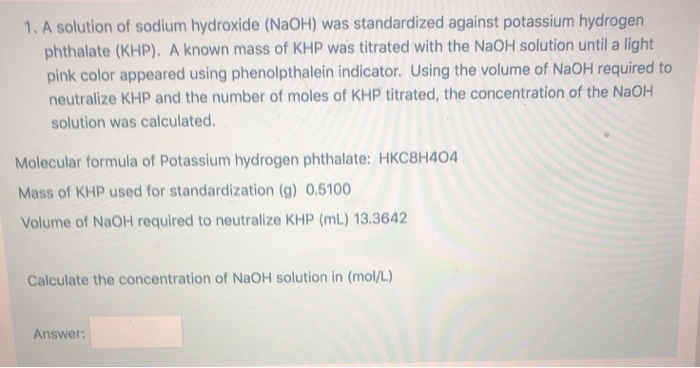1. A solution of sodium hydroxide (NaOH) was standardized against potassium hydrogen phthalate (KHP). A known mass of KHP was titrated with the NaOH solution until a light pink color appeared using phenolpthalein indicator. Using the volume of NaOH required to neutralize KHP and the number of moles of KHP titrated, the concentration of the NaOH solution was calculated. Molecular formula of Potassium hydrogen phthalate: HKC8H404 Mass of KHP used for standardization (g) 0.5100 Volume of NaOH required to neutralize...

• ### 1. If the bubble in the tip of the buret is not removed prior to performing...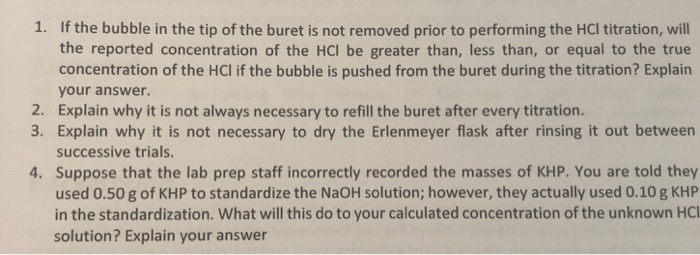1. If the bubble in the tip of the buret is not removed prior to performing the HCl titration, will the reported concentration of the HCl be greater than, less than, or equal to the true concentration of the HCl if the bubble is pushed from the buret during the titration? Explain your answer. 2. Explain why it is not always necessary to refill the buret after every titration. 3. Explain why it is not necessary to dry the Erlenmeyer...

• ### A. Preparation of Sodium Hydroxide Solution g g mass of Erlenmeyer flask + KHP 00 09...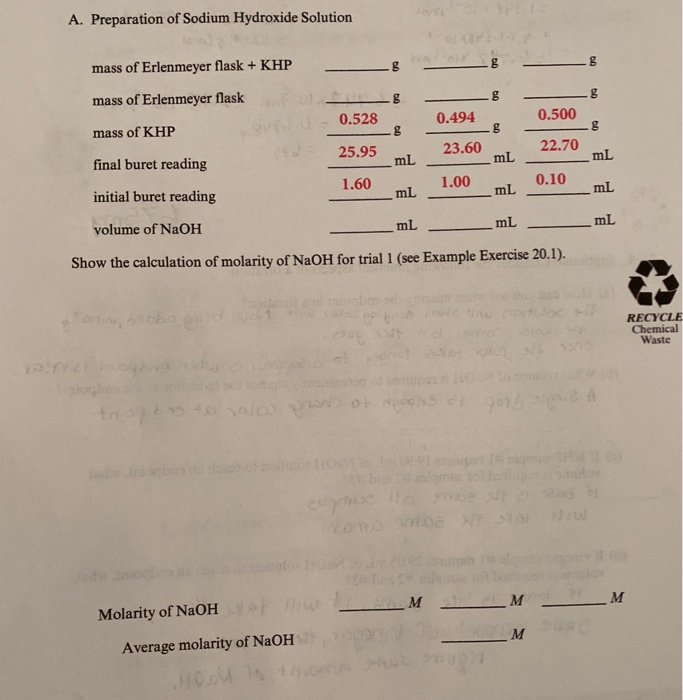A. Preparation of Sodium Hydroxide Solution g g mass of Erlenmeyer flask + KHP 00 09 mass of Erlenmeyer flask 0.494 0.500 mass of KHP LES 0.528 g 25.95 mL g 23.60 22.70 mL mL final buret reading 1.60 1.00 0.10 mL mL mL initial buret reading mL mL mL volume of NaOH Show the calculation of molarity of NaOH for trial 1 (see Example Exercise 20.1). 305 RECYCLE Chemical Waste volo Wo NON Omer _M M M Molarity of...

• ### 2. Calculate the mass of solid sodium hydroxide needed to prepare 300 mL of 0.1 M...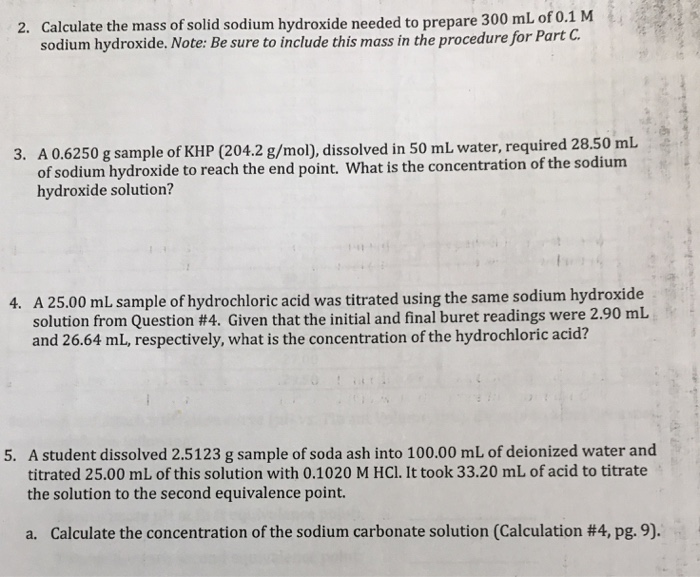2. Calculate the mass of solid sodium hydroxide needed to prepare 300 mL of 0.1 M sodium hydroxide. Note: Be sure to include this mass in the procedure for Part C. 3. A 0.6250 g sample of KHP (204.2 g/mol), dissolved in 50 mL water, required 28.50 mL of sodium hydroxide to reach the end point. What is the concentration of the sodium hydroxide solution? 4. A 25.00 mL sample of hydrochloric acid was titrated using the same sodium hydroxide...